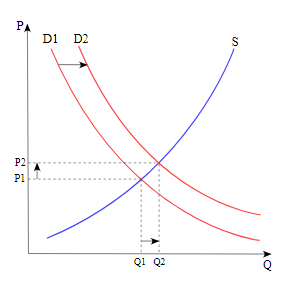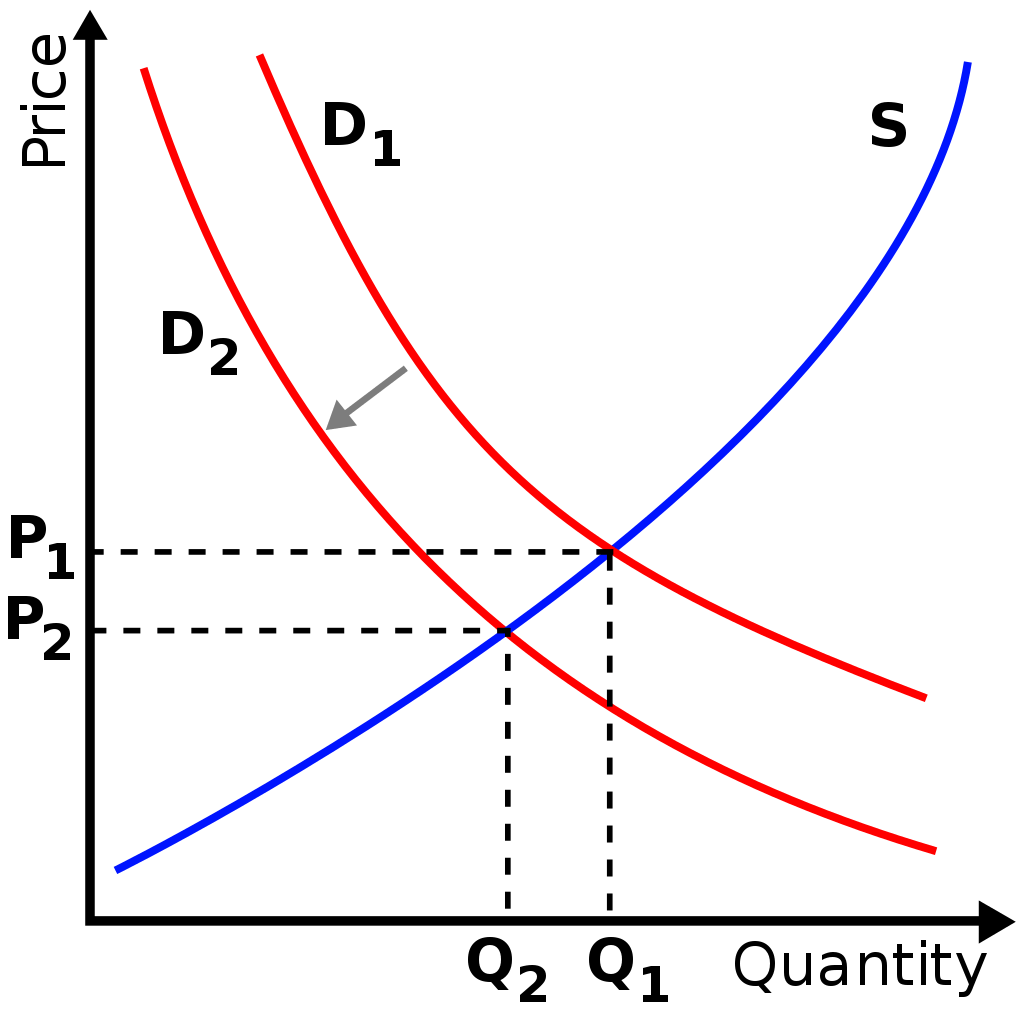# Shifts in Demand and Supply: Decrease and Increase, Concepts, Examples

The compilation of these Market Equilibrium Notes makes students exam preparation simpler and organised.

## Shifts in Demand and Supply

We often hear news about how prices of gold change every single moment. They never seem to be static and are always fluctuating. Thus a graphical representation of market equilibrium for gold would always keep changing. This can happen due to many factors that come under the umbrella of either shift or increase in demand, supply or both.

### The Shift in Demand and Supply

Definitely, if there is any change in supply, demand or both the market equilibrium would change. Let’s recollect the factors that induce changes in demand and supply:

Shift in Demand
The demand for a product changes due to an alteration in any of the following factors:

• Price of complementary goods
• Price of substitute goods
• Income
• Tastes and preferences
• An expectation of change in the price in future
• Population

Shift in Supply
The supply of product changes due to an alteration in any of the following factors:

• Prices of factors of production
• Prices of other goods
• State of technology
• Taxation policy
• An expectation of change in price in future
• Goals of the firm
• Number of firms

Now let us study individually how market equilibrium changes when only demand changes, only supply changes and when both demand and supply change.

### When only Demand Changes

A change in demand can be recorded as either an increase or a decrease. Note that in this case there is a shift in the demand curve.

Increase in Demand
When there is an increase in demand, with no change in supply, the demand curve tends to shift rightwards. As the demand increases, a condition of excess demand occurs at the old equilibrium price. This leads to an increase in competition among the buyers, which in turn pushes up the price.

Of course, as price increases, it serves as an incentive for suppliers to increase supply and also leads to a fall in demand. It is important to realize that these processes continue to operate until a new equilibrium is established. Effectively, there is an increase in both the equilibrium price and quantity.Decrease in Demand
Under conditions of a decrease in demand, with no change in supply, the demand curve shifts towards left. When demand decreases, a condition of excess supply is built at the old equilibrium level. This leads to an increase in competition among the sellers to sell their produce, which obviously decreases the price.

Now as for price decreases, more consumers start demanding the good or service. Observably, this decrease in price leads to a fall in supply and a rise in demand. This counter mechanism continues until the conditions of excess supply are wiped out at the old equilibrium level and a new equilibrium is established. Effectively, there is a decrease in both the equilibrium price and quantity.### When only Supply Changes

A change in supply can be noted as either an increase or a decrease. Note that in this case there is a shift in the supply curve.

Increase in Supply
When supply increases, accompanied by no change in demand, the supply curve shift towards the right. When supply increases, a condition of excess supply arises at the old equilibrium level. This induces competition among the sellers to sell their supply, which in turn decreases the price.

This decrease in price, in turn, leads to a fall in supply and a rise in demand. These processes operate until a new equilibrium level is attained. Lastly, such conditions are marked by a decrease in price and an increase in quantity.Decrease in Supply
When the supply decreases, accompanied by no change in demand, there is a leftward shift of the supply curve. As supply decreases, a condition of excess demand is created at the old equilibrium level. Effectively there is increased competition among the buyers, which obviously leads to a rise in the price.

An increase in price is accompanied by a decrease in demand and an increase in supply. This continues until a new equilibrium level is attained. Further, there is a rise in equilibrium price but a fall in equilibrium quantity.

### When both Demand and Supply Change

Generally, the market situation is more complex than the above-mentioned cases. That means, generally, supply and demand do not change in an individual manner. There is a simultaneous change in both entities. This gives birth to four cases:

1. Both Demand and Supply Decrease
2. Both Demand and Supply Increase
3. Demand Decreases But Supply Increases
4. Demand Increases But Supply Decreases

1. Both Demand and Supply Decrease
The final market conditions can be determined only by a deduction of the magnitude of the decrease in both demand and supply. In fact, both the demand and supply curve shift towards the left. Essentially, there is a need to compare their magnitudes. Such conditions are better analyzed by dividing this case further into three:

The decrease in demand = decrease in supply

When the magnitudes of the decrease in both demand and supply are equal, it leads to a proportionate shift of both the demand and supply curve. Consequently, the equilibrium price remains the same but there is a decrease in the equilibrium quantity.

The decrease in demand > decrease in supply

When the decrease in demand is greater than the decrease in supply, the demand curve shifts more towards the left relative to the supply curve. Effectively, there is a fall in both equilibrium quantity and price.

The decrease in demand < decrease in supply

In a case in which the decrease in demand is smaller than the decrease in supply, the leftward shift of the demand curve is less than the leftward shift of the supply curve. Notably, there is a rise in equilibrium price accompanied by a fall in equilibrium quantity.

Both Demand and Supply Increase
In such a condition both demand and supply shift rightwards. So, in order to study changes in market equilibrium, we need to compare the increase in both entities and then conclude accordingly. Such a condition is further studied better with the help of the following three cases:

The increase in demand = increase in supply

If the increase in both demand and supply is exactly equal, there occurs a proportionate shift in the demand and supply curve. Consequently, the equilibrium price remains the same. However, the equilibrium quantity rises.

The increase in demand > increase in supply

In such a case, the right shift of the demand curve is more relative to that of the supply curve. Effectively, both equilibrium price and quantity tend to increase.

The increase in demand < increase in supply

When the increase is demand is less than the increase in supply, the right shift of the demand curve is less than the right shift of supply curve. In this case, the equilibrium price falls whereas the equilibrium quantity rises.

Demand Decreases but Supply Increases
This condition translates to the fact that the demand curve shifts leftwards whereas the supply curve shifts rightwards. As they move in opposite directions, the final market conditions are deduced by pointing out the magnitude of their shifts. Here, three cases further arise which are as follows:

The decrease in demand = increase in supply

In this case, although the two curves move in opposite directions, the magnitudes of their shifts is effectively the same. As a result, the equilibrium quantity remains the same but the equilibrium price falls.

The decrease in demand > increase in supply

When the decrease in demand is greater than the increase in supply, the relative shift of the demand curve is proportionately more than the supply curve. Effectively, both the equilibrium quantity and price fall.

The decrease in demand < increase in supply

Here, the leftward shift of the demand curve is less than the rightward shift of the supply curve. It is important to realize, that the equilibrium quantity rises whereas the equilibrium price falls.

Demand Increases but Supply Decreases
Similar to the aforementioned condition, here also the demand and supply curve moves in the opposite directions. However, the demand curve shift towards the right (indicating an increase in demand), and the supply curve shift towards the left (indicating a decrease in supply). Further, this is studied with the help of the following three cases:

Increase in demand = decrease in supply

When the increase in demand is equal to the decrease in supply, the shifts in both supply and demand curves are proportionately equal. Effectively, the equilibrium quantity remains the same however the equilibrium price rises.

Increase in demand > decrease in supply

In this case, the right shift of the demand curve is proportionately more than the leftward shift of the supply curve. Hence, both equilibrium quantity and price rise.

Increase in demand < decrease in supply

If the increase in demand is less than the decrease in supply, the shift of the demand curve tends to be less than that of the supply curve. Effectively, equilibrium quantity falls whereas the equilibrium price rises.

Example:

Question:
Explain how market equilibrium changes when there is an increase only in the demand.